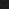JavaPerspective.com  >   Beginner Tutorials  >   5. Java in Practice  >   5.8. Java code exercises  >   5.8.1. Code exercise 1

5.8.1. Code exercise 1
Last updated: 27 January 2013.

Write a method named sumOfMultiplesOfFive that returns the sum of integer multiples of five, ranging from 0 to 100 included:

0 + 5 + 10 + 15 + ... + 100

public final class Practice {

public int sumOfMultiplesOfFive(){

// the code goes here

}

}

public final class Practice {

public int sumOfMultiplesOfFive(){

int result = 0;

for(int i=0; i<=100; i=i+5)

result = result + i;

return result;

}

}

You are here :  JavaPerspective.com  >   Beginner Tutorials  >   5. Java in Practice  >   5.8. Java code exercises  >   5.8.1. Code exercise 1
Next tutorial :  JavaPerspective.com  >   Beginner Tutorials  >   5. Java in Practice  >   5.8. Java code exercises  >   5.8.2. Code exercise 2